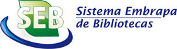Autor
 Palavra-chave
 Tipo do documento
 Ano
 País
 Idioma
 Ordenar por: Relevância Autor Título AnoPrimeira ... 1 ... ÚltimaFreese, Jeremy. This article presents a method and program for identifying poorly fitting observations for maximum-likelihood regression models for categorical dependent variables. After estimating a model, the program least likely will list the observations that have the lowest predicted probabilities of observing the value of the outcome category that was actually observed. For example, when run after estimating a binary logistic regression model, least likely will list the observations with a positive outcome that had the lowest predicted probabilities of a positive outcome and the observations with a negative outcome that had the lowest predicted probabilities of a negative outcome. These can be considered the observations in which the outcome is most surprising given... Tipo: Journal Article Palavras-chave: Outliers; Predicted probabilities; Categorical dependent variables; Logistic regression; Research Methods/ Statistical Methods. Ano: 2002 URL: http://purl.umn.edu/116014Long, J. Scott; Freese, Jeremy. The post-estimation command prcounts for generating predicted probabilities after using poisson, nbreg, zip, and zinb is introduced and illustrated. Tipo: Journal Article Palavras-chave: Predicted probabilities; Count models; Research Methods/ Statistical Methods. Ano: 2001 URL: http://purl.umn.edu/115935Mitchell, Michael N.; Chen, Xiao. This paper considers the role of covariates when using predicted probabilities to interpret main effects and interactions in logit models. While predicted probabilities are very intuitive for interpreting main effects and interactions, the pattern of results depends on the contribution of covariates. We introduce a concept called the covariate contribution, which reflects the aggregate contribution of all of the remaining predictors (covariates) in the model and a family of tools to help visualize the relationship between predictors and the predicted probabilities across a variety of covariate contributions. We believe this strategy and the accompanying tools can help researchers who wish to use predicted probabilities as an interpretive framework for... Tipo: Journal Article Palavras-chave: Logistic regression; Predicted probabilities; Main effects; Interactions; Covariate contribution; Research Methods/ Statistical Methods. Ano: 2005 URL: http://purl.umn.edu/117500